Question

Find the equivalent resistance between points A and B in the drawing.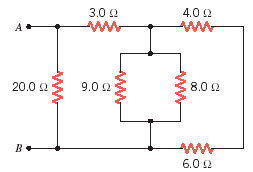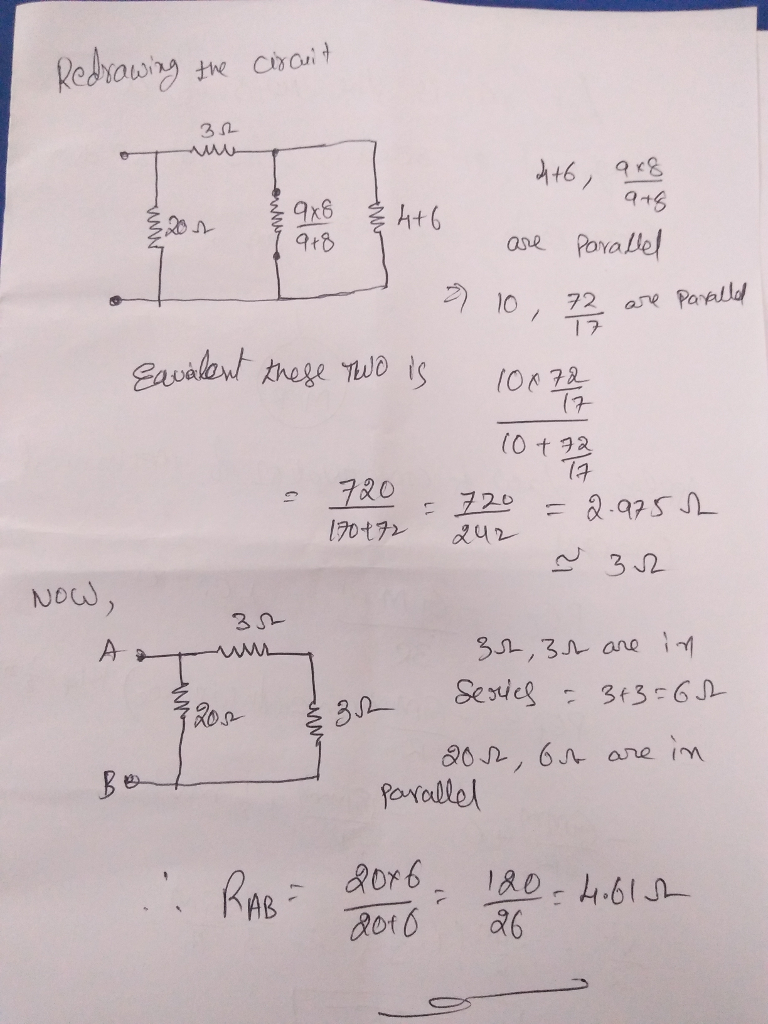#### Earn Coins

Coins can be redeemed for fabulous gifts.

Similar Homework Help Questions
• ### Find the equivalent resistance between points A and B in the drawing. 3.0 Ω 4.0 2...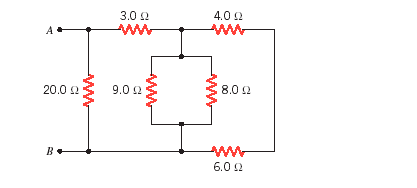Find the equivalent resistance between points A and B in the drawing. 3.0 Ω 4.0 2 8.0 Ω 20012 9.0 12 6.0 Ω

• ### 4. Find the equivalent resistance between points A and B in the drawing (R1 = 4.0...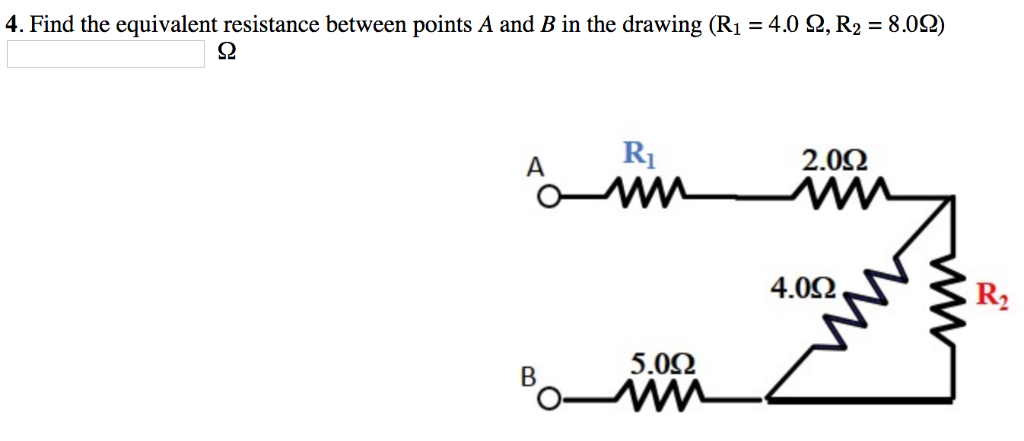4. Find the equivalent resistance between points A and B in the drawing (R1 = 4.0 Ω, R2 = 8.0Ω) S2 2.0Ω 4.0Ω R2 5.0Ω

• ### Resistors 3.0 Ω 4.0 Ω a. 4) a) Determine the equiv. resistance of the network at...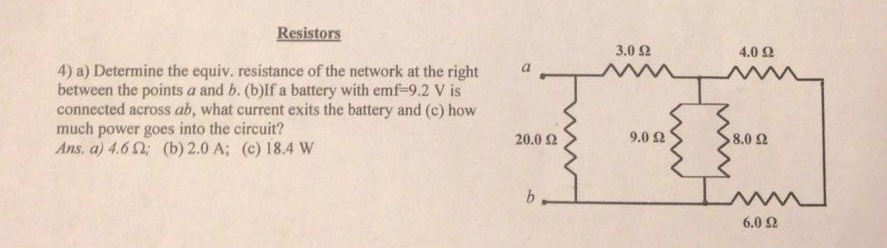Resistors 3.0 Ω 4.0 Ω a. 4) a) Determine the equiv. resistance of the network at the right between the points a and b. (b)If a battery with emf 9.2 V is connected across ab, what current exits the battery and (c) how much power goes into the circuit? Ans. a) 4.6 2; (b) 2.0 A; (c) 18.4 W 20.0 Ω 9.0 Ω 8.0 2 6.0 Ω

• ### Find the equivalent resistance between points A and B in the drawing. Find the equivalent resistance...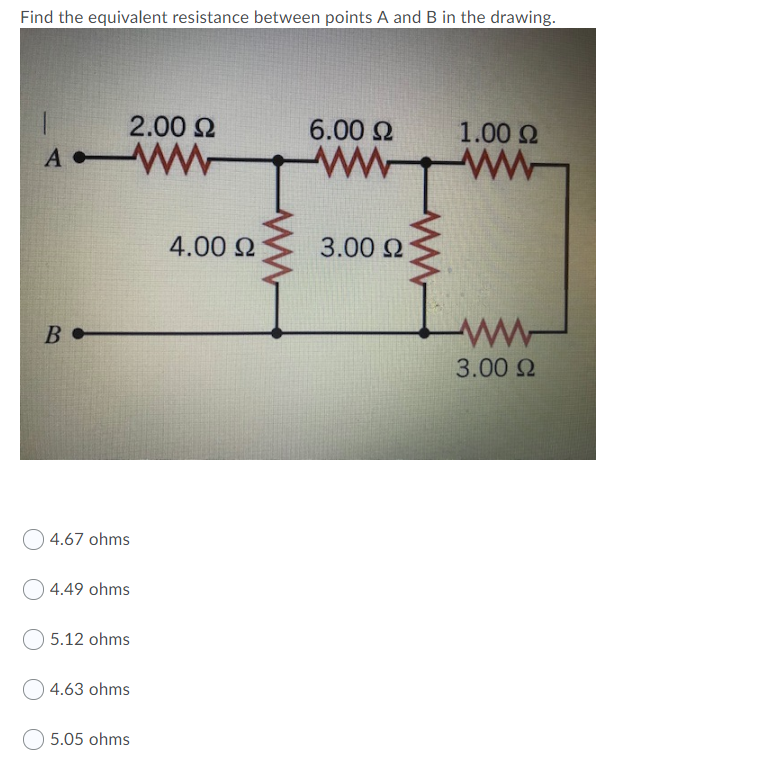Find the equivalent resistance between points A and B in the drawing. Find the equivalent resistance between points A and B in the drawing. 2.00 Ω 6.00 Ω 1.00 Ω A 4.00 Ω 3.00 Ω B - ΑΛΛ- 3.00 Ω 4.67 ohms Ο4.49 ohms 5.12 ohms 4.63 ohms 5.05 ohms

• ### 9. Determine the equivalent resistance between the points A and B for the group of resistors...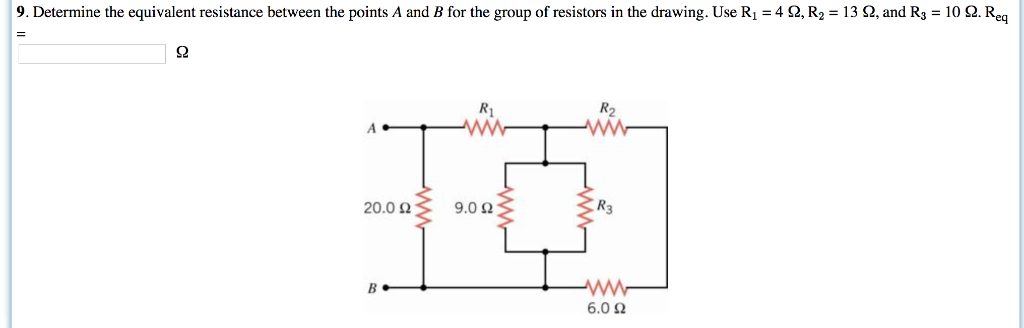9. Determine the equivalent resistance between the points A and B for the group of resistors in the drawing. Use R1-4 Ω, R2 = 13 Ω and R3 = 10 Ω Re Ri R2 20.0 Ω 9.0 Ω R3 6.0 Ω

• ### Find the equivalent resistance between points A and B in the drawing. Number____ Units____

Find the equivalent resistance between points A and B in the drawing. Number____ Units____

• ### (a) Find the equivalent resistance between points a and b in Figure P18.2. (b) Calculate the...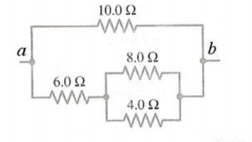(a) Find the equivalent resistance between points a and b in Figure P18.2. (b) Calculate the current in each resistor if a potential difference of 34.0 V is applied between points a and b. 10.0 Ω 8.0 Ω 4.0 Ω

• ### 65) Determine the equivalent resistance between the points A and B for the group of resistors...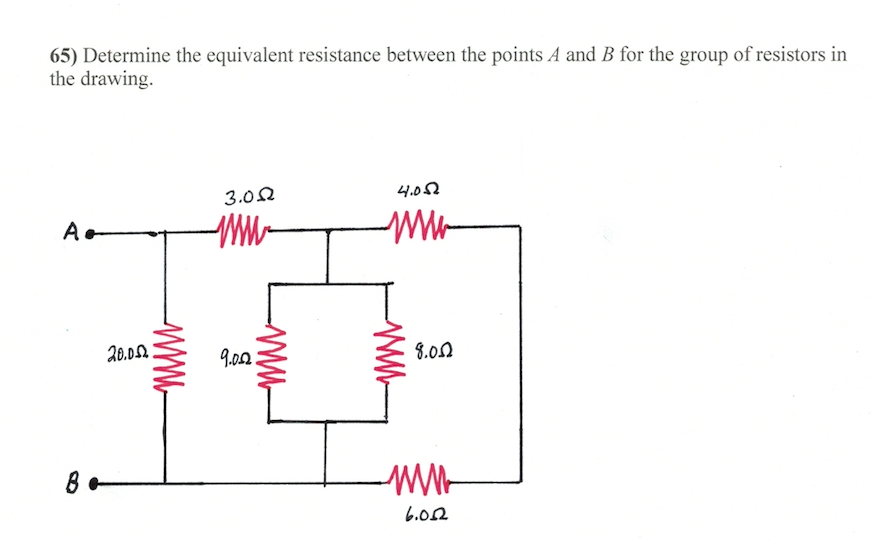65) Determine the equivalent resistance between the points A and B for the group of resistors in the drawing. 3.0 Ω 4.0 Ω Xo.DL 9.0Ω 3,0Ω .02

• ### Find the equivalent resistance between points A and B in the drawing. RI 26 Ω R2...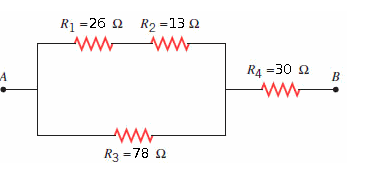Find the equivalent resistance between points A and B in the drawing. RI 26 Ω R2 1362 R4-30 Ω Ra 78 Ω